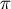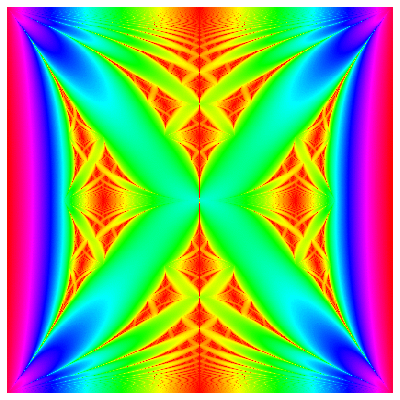Fall 2003

# Mathematics Math21b Fall 2003

## Linear Algebra and Differential Equations

Office: SciCtr 434
Email: knill@math.harvard.eduThe picture below illustrates a function f(x,y) of two variables which is defined using determinant of matrices. For rational y=p/q form f(x,y) = log[ | det (L(y) -x) | ]/q, where L(y) is a (q x q) matrix with side diagonal entries 1 and diagonal entries V(k) = 2 cos(2k p/q ):
 ``` | V(1) 1 0 ... 0 1 | | 1 V(2) 1 ... ... 0 | L(y)= | 0 1 ... ... ... ... | | ... ... ... ... 1 0 | | 0 ... ... 1 V(q-1) 1 | | 1 0 ... 0 1 V(q) | ```